# 梯度下降法

$x_{n+1} = x_{n} - \gamma\frac{d}{dx}f(x_n)$

$x_{n+1} = x_{n} - \gamma\frac{f(x_{n+1})-f(x_n)}{x_{n+1}-x_{n}}$

$\frac{d}{dx}f(x_n)=0$

# 针对梯度下降算法的改进

## 衰减参数的引入

1. 由于设置的学习率太小，导致一直出于下降优化过程，但是直到达到了最大迭代次数，也没能优化到最优值。如下图所示，正因为学习率设置的太低而导致迭代过程无法收敛。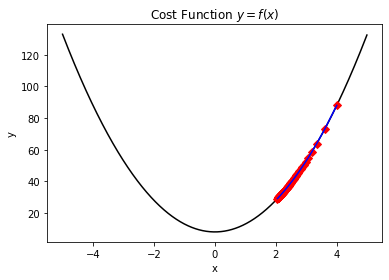2. 由于设置的学习率太大，导致出现"震荡"现象，同样无法尽快优化到收敛值。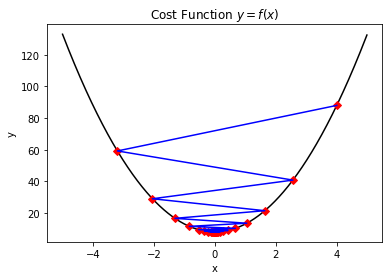$x_{n+1} = x_{n} - \frac{\gamma}{n+1}\frac{f(x_{n+1})-f(x_n)}{x_{n+1}-x_{n}}$

import matplotlib.pyplot as plt
x = [i for i in range(100)]
y = [1/(i + 1) for i in range(100)]
plt.figure()
plt.plot(x,y,color='black')
plt.title('Decay parameter $\gamma$')
plt.xlabel('n')
plt.ylabel('$\gamma$')
plt.show()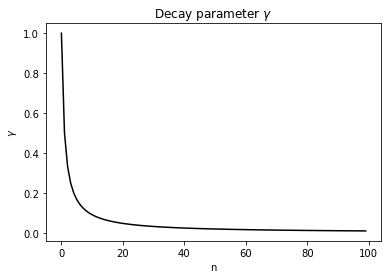## 冲量参数的引入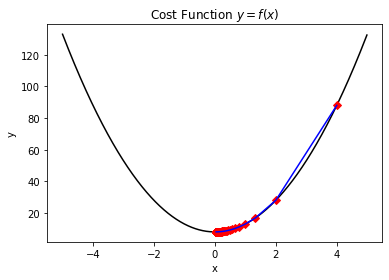$Ft=m\frac{d}{dt}x_1-m\frac{d}{dt}x_0$

$x_{n+1} = x_{n} - \frac{\gamma}{n+1}\frac{f(x_{n+1})-f(x_n)}{x_{n+1}-x_{n}} + m(x_{n}-x_{n-1})$

# 定义代价函数

$f(x)=5x^2+8$

import matplotlib.pyplot as plt

def cost_function(x):
return 5 * x ** 2 + 8

plt.figure()
x = [i / 100 - 5 for i in range(1000)]
y = [cost_function(i) for i in x]
plt.plot(x, y, color = 'black')
plt.title('Cost Function $y = f(x)$') # Latex Type Equation.
plt.xlabel('x')
plt.ylabel('y')
plt.show()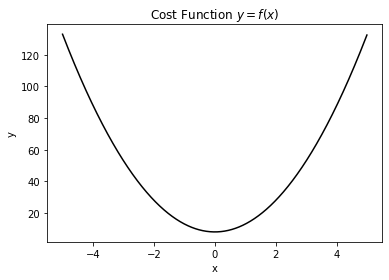# 梯度下降法的代码实现

from scipy.optimize import minimize as scipy_minimize
from tqdm import trange
import matplotlib.pyplot as plt
import numpy as np

DELTA = 1e-06
EPSILON = 1e-06
MAX_STEPS = 100
GAMMA = 0.6
MOMENTUM =0.2

def cost_function(x):
return 5 * x ** 2 + 8

def gradient(x, func):
return (func(x + DELTA) - func(x)) / DELTA

def next_x(x, func, iterations, v):
_tmpx = x - GAMMA  * gradient(x, func) / (iterations + 1) + MOMENTUM * v
return _tmpx if cost_function(_tmpx) < cost_function(x) and gradient(_tmpx, func) * gradient(x, func) >= 0 else x

def minimize(x0, func):
_x1 = x0
_y1 = func(_x1)
plot_x = [_x1]
plot_y = [_y1]
v = 0
for i in trange(MAX_STEPS):
_x = next_x(_x1, func, i, v)
_y = func(_x)
v = _x - _x1
if v == 0:
continue
if abs(_y - _y1) <= EPSILON:
print ('The minimum value {} founded :)'.format(_y))
print ('The correspond x value is: {}'.format(_x))
print ('The iteration times is: {}'.format(i))
return _y, _x, plot_y, plot_x
_x1 = _x
_y1 = _y
plot_x.append(_x1)
plot_y.append(_y1)
print ('The last value of y is: {}'.format(_y))
print ('The last value of x is: {}'.format(_x))
return _y, _x, plot_y, plot_x

if __name__ == '__main__':
x0 = 4
yt, xt, py, px = minimize(x0, cost_function)
plt.figure()
x = [i / 100 - 5 for i in range(1000)]
y = [cost_function(i) for i in x]
plt.plot(x, y, color = 'black')
plt.plot(px, py, 'D', color = 'red')
print ('The function evaluation times is: {}'.format(len(py)))
plt.plot(px, py, color = 'blue')
plt.title('Cost Function $y = f(x)$') # Latex Type Equation.
plt.xlabel('x')
plt.ylabel('y')
plt.show()
result = scipy_minimize(cost_function, x0, method='BFGS', options={'disp':True})
print ('The scipy method founded x is: {}'.format(result.x))
print ('The correspond cost function value is: {}'.format(cost_function(result.x)))


The last value of y is: 8.00000000000125
The last value of x is: -5.000249774511634e-07
The function evaluation times is: 4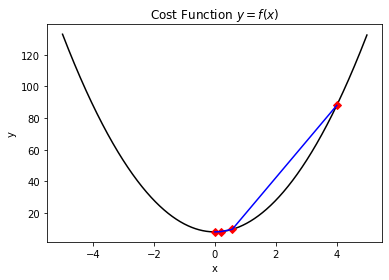Optimization terminated successfully.
Current function value: 8.000000
Iterations: 3
Function evaluations: 8
Gradient evaluations: 4
The scipy method founded x is: [-9.56720569e-09]
The correspond cost function value is: [8.]


# 补充测试案例

from scipy.optimize import minimize as scipy_minimize
from tqdm import trange
import matplotlib.pyplot as plt
import numpy as np

DELTA = 1e-06
EPSILON = 1e-06
MAX_STEPS = 100
GAMMA = 2
MOMENTUM =0.9

def cost_function(x):
return np.sin(x)

def gradient(x, func):
return (func(x + DELTA) - func(x)) / DELTA

def next_x(x, func, iterations, v):
_tmpx = x - GAMMA  * gradient(x, func) / (iterations + 1) + MOMENTUM * v
return _tmpx if cost_function(_tmpx) < cost_function(x) and gradient(_tmpx, func) * gradient(x, func) >= 0 else x

def minimize(x0, func):
_x1 = x0
_y1 = func(_x1)
plot_x = [_x1]
plot_y = [_y1]
v = 0
for i in trange(MAX_STEPS):
_x = next_x(_x1, func, i, v)
_y = func(_x)
v = _x - _x1
if v == 0:
continue
if abs(_y - _y1) <= EPSILON:
print ('The minimum value {} founded :)'.format(_y))
print ('The correspond x value is: {}'.format(_x))
print ('The iteration times is: {}'.format(i))
return _y, _x, plot_y, plot_x
_x1 = _x
_y1 = _y
plot_x.append(_x1)
plot_y.append(_y1)
print ('The last value of y is: {}'.format(_y))
print ('The last value of x is: {}'.format(_x))
return _y, _x, plot_y, plot_x

if __name__ == '__main__':
x0 = 1.4
yt, xt, py, px = minimize(x0, cost_function)
plt.figure()
x = [i / 100 - 5 for i in range(1000)]
y = [cost_function(i) for i in x]
plt.plot(x, y, color = 'black')
plt.plot(px, py, 'D', color = 'red')
print ('The function evaluation times is: {}'.format(len(py)))
plt.plot(px, py, color = 'blue')
plt.title('Cost Function $y = f(x)$') # Latex Type Equation.
plt.xlabel('x')
plt.ylabel('y')
plt.show()
result = scipy_minimize(cost_function, x0, method='BFGS', options={'disp':True})
print ('The scipy method founded x is: {}'.format(result.x))
print ('The correspond cost function value is: {}'.format(cost_function(result.x)))


The minimum value -0.9999986210818882 founded :)
The correspond x value is: -1.569135653179335
The iteration times is: 14
The function evaluation times is: 11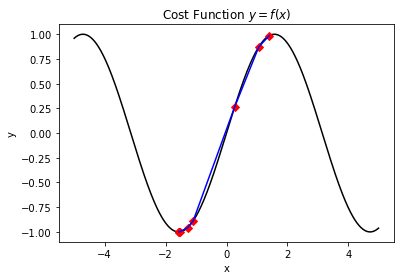Optimization terminated successfully.
Current function value: -1.000000
Iterations: 2
Function evaluations: 16
Gradient evaluations: 8
The scipy method founded x is: [-1.57079993]
The correspond cost function value is: [-1.]


# 版权声明

posted @ 2021-01-31 00:00  DECHIN  阅读(212)  评论(0编辑  收藏  举报